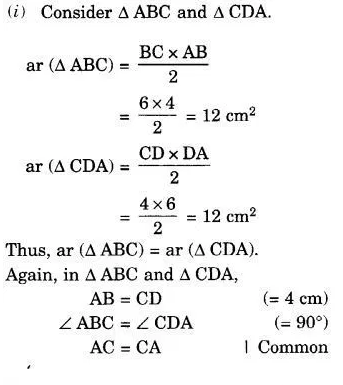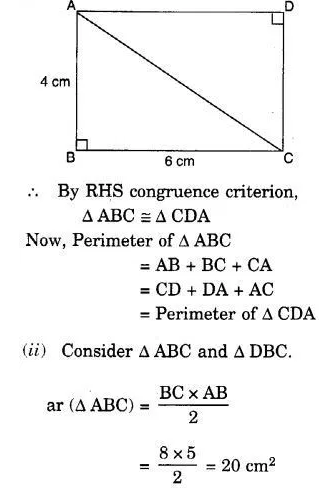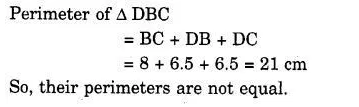# In a squared sheet, draw two triangles of equal areas such that

In a squared sheet, draw two triangles of equal areas such that

1. the triangles are congruent
2. the triangles are not congruent. What can you say about their perimeters?